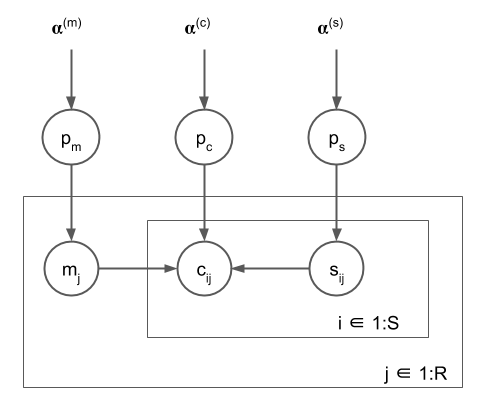# Subsampling not applied to all sites?

I’m having trouble getting subsampling working for a model with nested plates. Model, MWE, and error below. `m`, `c_ij`, and `s_ij` are all binary (but set as Categorical to allow for future expansion of the domains). Their CPDs (the `p` variables) are all drawn from a currently uninformative Dirichlet prior. The observed variables are `m`, `c`, and `s` (for machine, metric, sample).

Initially, if I leave out subsampling then SVI runs fine, and I’m able to recover the correct CPDs for `p_m` and `p_s` (based on training data I simulate; not shown here), although I can’t yet correctly recover `p_c`, so I suspect something is off with indexing into that CPD on the `c` sample line.

Additionally, and perhaps more simply, when I do try to use subsampling on the inner plate, I get a dimension mismatch at site `c` error. Looking at the shape trace it seems the value at `s` is not properly getting subsampled, but its distribution is. This is odd, because if I examine the shapes of the variables returned by `m, s, c = model(S, R)` they all look to be correctly subsampled.

Any idea why this is happening? I’m hoping that fixing this subsampling error will in turn allow the model to properly recover `p_c`. Thanks!MWE:

``````import numpy as np
import torch
from torch.distributions import constraints
from pyro.ops.indexing import Vindex
import pyro.optim
import pyro.infer
import pyro.distributions as dist

R = 20
S = 100
subsample_size = 10

def model(S, R):

run_plate = pyro.plate('run_plate', R, dim=-1)
sample_plate = pyro.plate('sample_plate', S, dim=-2, subsample_size=subsample_size)

# global CPDs
p_m = pyro.sample('p_m', dist.Dirichlet(torch.ones(2)))
p_s = pyro.sample('p_s', dist.Dirichlet(torch.ones(2)))
with pyro.plate_stack("c_plate", (2,2)):
p_c = pyro.sample('p_c', dist.Dirichlet(torch.ones(2,2,2)))

# data
with run_plate:
# machine
m = pyro.sample('m', dist.Categorical(p_m))

# sample and metric
with sample_plate:
s = pyro.sample('s', dist.Categorical(p_s))
c = pyro.sample('c', dist.Categorical(Vindex(p_c)[m, s, :])) # (machine, sample, metric)

return m, s, c

def guide(S, R):

# machine
m_prior = pyro.param('m_post', machine_prior_t, constraint=constraints.positive)
p_m = pyro.sample('p_m', dist.Dirichlet(m_prior))

# sample
s_prior = pyro.param('s_post', sample_prior_t, constraint=constraints.positive)
p_s = pyro.sample('p_s', dist.Dirichlet(s_prior))

# metric
c_prior = pyro.param('c_post', metric_prior_t, constraint=constraints.positive)  # (machine, sample, metric)
with pyro.plate_stack("c_plate", (2,2)):
p_c = pyro.sample('p_c', dist.Dirichlet(c_prior))

# condition model on dummy MWE data
observations = {'m': torch.ones(R, dtype=torch.long),
's': torch.ones((S, R), dtype=torch.long),
'c': torch.ones((S, R), dtype=torch.long),
}
cond_model = pyro.condition(model, data=observations)

# run svi
svi = pyro.infer.SVI(cond_model, guide, pyro.optim.Adam({"lr": .0001}), loss=pyro.infer.Trace_ELBO())
for step in range(10000):
svi.step(S, R)
``````

Error:

``````ValueError: Shape mismatch inside plate('sample_plate') at site c dim -2, 10 vs 100
Trace Shapes:
Param Sites:
Sample Sites:
run_plate dist        |
value     20 |
sample_plate dist        |
value     10 |
p_m dist        | 2
value        | 2
p_s dist        | 2
value        | 2
c_plate_0 dist        |
value      2 |
c_plate_1 dist        |
value      2 |
p_c dist   2  2 | 2
value   2  2 | 2
m dist     20 |
value     20 |
s dist  10 20 |
value 100 20 |``````

I was able to fix the shape issue by changing to the paradigm of including the `obs` keyword in `sample` sites, and then subsample indexing into that.

I think there’s still an indexing issue somewhere as I’m not able to correctly recover `p_c`, so if anyone spots it that’d be super helpful. I’m still not clear on the `Vindex` usage.

``````import numpy as np
import torch
from torch.distributions import constraints
from pyro.ops.indexing import Vindex
import pyro.optim
import pyro.infer
import pyro.distributions as dist

R = 20
S = 100
subsample_size = 10

observations = {'m': torch.ones(R, dtype=torch.long),
's': torch.ones((S, R), dtype=torch.long),
'c': torch.ones((S, R), dtype=torch.long),
}

def model(S, R):

run_plate = pyro.plate('run_plate', R, dim=-1)
sample_plate = pyro.plate('sample_plate', S, dim=-2, subsample_size=subsample_size)

# global CPDs
p_m = pyro.sample('p_m', dist.Dirichlet(torch.ones(2)))
p_s = pyro.sample('p_s', dist.Dirichlet(torch.ones(2)))
with pyro.plate_stack("c_plate", (2,2)):
p_c = pyro.sample('p_c', dist.Dirichlet(torch.ones(2,2,2)))

# data
with run_plate:
# machine
m = pyro.sample('m', dist.Categorical(p_m), obs=observations['m'])

# sample and metric
with sample_plate as ind:
s = pyro.sample('s', dist.Categorical(p_s), obs=observations['s'][ind])
c = pyro.sample('c', dist.Categorical(Vindex(p_c)[m, s, :]), obs=observations['c'][ind]) # (machine, sample, metric)

def guide(S, R):

# machine
m_prior = pyro.param('m_post', machine_prior_t, constraint=constraints.positive)
p_m = pyro.sample('p_m', dist.Dirichlet(m_prior))

# sample
s_prior = pyro.param('s_post', sample_prior_t, constraint=constraints.positive)
p_s = pyro.sample('p_s', dist.Dirichlet(s_prior))

# metric
c_prior = pyro.param('c_post', metric_prior_t, constraint=constraints.positive)  # (machine, sample, metric)
with pyro.plate_stack("c_plate", (2,2)):
p_c = pyro.sample('p_c', dist.Dirichlet(c_prior))

# run svi
svi = pyro.infer.SVI(model, guide, pyro.optim.Adam({"lr": .0001}), loss=pyro.infer.Trace_ELBO())
for step in range(10000):
svi.step(S, R)``````

Solved the indexing issue too. Just needed to swap the dimensions in `run_plate` and `sample_plate` to match the corresponding variables (`m` and `s`) used for indexing into `p_c` at the `c` sample site.

``````import numpy as np
import torch
from torch.distributions import constraints
from pyro.ops.indexing import Vindex
import pyro.optim
import pyro.infer
import pyro.distributions as dist

R = 20
S = 100
subsample_size = 10

observations = {'m': torch.ones(R, dtype=torch.long),
's': torch.ones((S, R), dtype=torch.long),
'c': torch.ones((S, R), dtype=torch.long),
}

def model(S, R):

run_plate = pyro.plate('run_plate', R, dim=-2)
sample_plate = pyro.plate('sample_plate', S, dim=-1, subsample_size=subsample_size)

# global CPDs
p_m = pyro.sample('p_m', dist.Dirichlet(torch.ones(2)))
p_s = pyro.sample('p_s', dist.Dirichlet(torch.ones(2)))
with pyro.plate_stack("c_plate", (2,2)):
p_c = pyro.sample('p_c', dist.Dirichlet(torch.ones(2,2,2)))

# data
with run_plate:
# machine
m = pyro.sample('m', dist.Categorical(p_m), obs=observations['m'])

# sample and metric
with sample_plate as ind:
s = pyro.sample('s', dist.Categorical(p_s), obs=observations['s'][ind])
c = pyro.sample('c', dist.Categorical(Vindex(p_c)[m, s, :]), obs=observations['c'][ind]) # (machine, sample, metric)

def guide(S, R):

# machine
m_prior = pyro.param('m_post', machine_prior_t, constraint=constraints.positive)
p_m = pyro.sample('p_m', dist.Dirichlet(m_prior))

# sample
s_prior = pyro.param('s_post', sample_prior_t, constraint=constraints.positive)
p_s = pyro.sample('p_s', dist.Dirichlet(s_prior))

# metric
c_prior = pyro.param('c_post', metric_prior_t, constraint=constraints.positive)  # (machine, sample, metric)
with pyro.plate_stack("c_plate", (2,2)):
p_c = pyro.sample('p_c', dist.Dirichlet(c_prior))

# run svi
svi = pyro.infer.SVI(model, guide, pyro.optim.Adam({"lr": .0001}), loss=pyro.infer.Trace_ELBO())
for step in range(10000):
svi.step(S, R)``````

@gbernstein FYI in the upcoming Pyro 1.3.0 release, we’ve added a `pyro.subsample` primitive that should make this much easier: just wrap any tensor in `pyro.subsample` within one or more subsampling `plate`s and it will be subsampled accordingly, without you having to manually index things.

1 Like